# Wodzicki residue

non-commutative residue

In algebraic quantum field theory (cf. also Quantum field theory), in order to write down an action in operator language one needs a functional that replaces integration [a1]. For the Yang–Mills theory (cf. Yang–Mills field) this is the Dixmier trace, which is the unique extension of the usual trace to the idealof the compact operatorssuch that the partial sums of its spectrum diverge logarithmically as the number of terms in the sum. The Wodzicki (or non-commutative) residue [a3] is the only extension of the Dixmier trace to the class of pseudo-differential operators (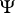DOs; cf. Pseudo-differential operator) which are not in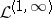. It is the only trace one can define in the algebra ofDOs (up to a multiplicative constant), its definition being:, withthe Laplace operator. It satisfies the trace condition: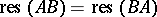. A very important property is that it can be expressed as an integral (local form):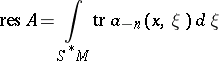with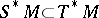the co-sphere bundle on(some authors put a coefficient in front of the integral, this gives the Adler–Manin residue).

If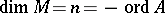(a compact Riemannian manifold,an elliptic operator,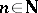), it coincides with the Dixmier trace, and one has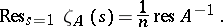The Wodzicki residue continues to make sense forDOs of arbitrary order and, even if the symbols,, are not invariant under coordinate choice, their integral is, and defines a trace. All residues at poles of the zeta-function of aDO can be easily obtained from the Wodzicki residue [a2].

How to Cite This Entry:
Wodzicki residue. Encyclopedia of Mathematics. URL: http://encyclopediaofmath.org/index.php?title=Wodzicki_residue&oldid=24145
This article was adapted from an original article by E. Elizalde (originator), which appeared in Encyclopedia of Mathematics - ISBN 1402006098. See original article